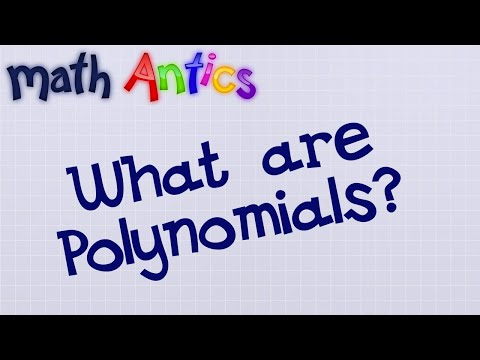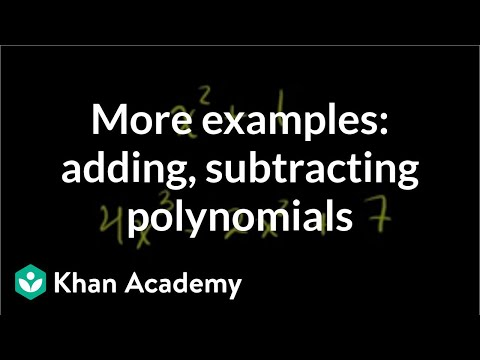## Polynomials

Subject: Optional Mathematics#### Overview

The collection of numbers and alphabets linked by the sign of multiplication and division is called algebraic term, 3, x, 5x, 9xy, $\fra{2x^2}{4y^2}$, etc. are some examples of the algebraic term. The term with no variable is called constant term. The number or alphabets by which variable is multiplied is called the coefficient of the term. 3 is coefficient in 3x. The number used for the coefficient is called literal coefficient.

### Polynomial

The collection of numbers and alphabets linked by the sign of multiplication and division is called algebraic term. 3, x, 5x, 9xy, $\frac{2x^2}{4y^2}$, etc are some examples of an algebraic term. The term with no variable is called constant term. The number or alphabets by which variable is multiplied is called the coefficient of the term. 3 is a coefficient of 3x. The number used for the coefficient is called numerical coefficient and alphabet used for coefficients are called literal coefficients. In the term 5ax,5 is numerical coefficient, 'a' is a literal coefficient and 'x' is avariable. In 7x3 , 7 is coefficient, x is base and 3 is power.

#### Standard form of Polynomial

f(x) =anxn + an-1 xn-1 + .........................+ ao
or. f(x) = ao + a1x+a2x + ............................ + anxn1. If an≠ 0, then n is called the degree of the polynomial f(x). The degree of the polynomial can never be negative.
2. anxn, an-1 xn-1,....................... ao are called the terms of the polynomial f(x). a is called the constant term.
3. aoa1,a2, ....... and are called the coefficients of the polynomial f(x).
4. If all an ≠ 0, then anxnis called the leading term and is called the leading coefficient of the polynomial.
5. If all the coefficient an, a1, ..... a are zero, then f(x) is called zero polynomial. The degree of zero polynomial is never defined.
6. The degree of a polynomial is zero if and only if it is a non-zero constant polynomial. f(x) =5 is a zero degree polynomial.

#### Equal polynomials

Two polynomial are said to be equal if and only if

1. Their degrees are same
2. Coefficients of corresponding terms are same.

If f(x) = anxn + an-1xn-1+ .............ao and g(x) = bmxm+bm-1xm-1 + ....................+ bo are equal polynomials, then n=m,an = bm.
an-1 = bm-1, ......ao = bo.
If polynomials f(x) and g(x) are equal, then we write f(x) =g(x).
Example :
If f(x) = 3x2+2x+1 and g(x) = $\frac{6}{2}$x2+$\frac{4}{2}$x+ $\frac{2}{2}$ then f(x) = g(x)

#### Addition and Subtraction of polynomials

Let f(x)and g(x) be two polynomials given as follows:
f(x) = a0 +a1x+a2 x2 +.....+ anxn
g(x) =b0+ b1x+b2x2+..........+bnxn
Then, their sum is defined as
f(x) = (a0+b0) + (a1+b1)x + (a2+b2)x2+ ........+(an+bn)xn.

Thus, the sum of two polynomials can be found by grouping the power terms, retaining their signs and adding the coefficients of like power.

For the calculation of sum or difference of polynomials, the polynomials are first kept in standard form. Then, the coefficients of like terms are added for sum and subtracted for the difference.

#### Properties of addition of polynomials

1. Closure property
If the sum of two polynomials over a set is again a polynomial over the same set, then polynomials are said to be closed under addition.
The sum of two polynomials over real numbers is also a polynomial over real numbers are closed under addition.
It means, the sum of two polynomials is again a polynomial.

2. Commutative property
For ant two polynomials f(x) and g(x),
f(x) + g(x) = g(x)+f(x)
This property is called the commutative property of addition.

3. Associative property
For any three polynomials f(x), g(x) and h(x) f(x) + {g(x) + h(x)} = {f(x) + g(x)} + h(x).
This property is called the associative property of addition.

For any polynomial f(x), there is a polynomial 0 such that.
f(x)+0 = f(x)
Then 0 is called additive identity.

For any polynomial f(x) there is a polynomial - f(x) such that
f(x) + {-f(x)} =0, the additive identity. Then -f(x) is called additive inverse of f(x).
##### Things to remember
• If the divisor is x+2, then a = -2
• If  the divisor is 2x-3, then a =$\frac{3}{2}$
• If the divisor is 2x+3, then a = $\frac{-3}{2}$
• If a polynomial p(x) is divided by x-a, then the remainder is p(a).
• It includes every relationship which established among the people.
• There can be more than one community in a society. Community smaller than society.
• It is a network of social relationships which cannot see or touched.
• common interests and common objectives are not necessary for society.
##### Videos for Polynomials##### Algebra Basics: What Are Polynomials? - Math Antics mathantics mathantics##### More examples of addition and subtraction of polynomials | Algebra II | Khan Academy

Here,

Given expressions:3x3+4

This is the polynomial because in3x3, exponent of x=3 is a whole number.

Here,

Given expressions$\frac{7}{3}$x4is a polynomials because exponent of x=4 is a whole number.

Here,

Given expressions$\frac{7}{3}$x4is a polynomials because exponent of x=4 is a whole number.

Here,

Given expressions:5

Here,5=5+0.x+0.x2.+......+0.x4 can be written.So it is a polynomial.

Here,

Given expression:√5.

√5=√5+0.x+0.x2+......+0.x4can be written.So it is polnomial.

Here,

Given expressions =-0.6x

Here numerical coefficient of x =-0.6.Ans.

Here,given ,x in 3xy.

here,3xy=(3y)x

∴Lateral coefficient of x=y.Ans.

Here,

Given ,5xy=(5x)y

∴ Lateral coefficient of y2=x.Ans.

Here given,$\frac{xy}{10}$=($\frac{x}{10}$)y

∴ coefficient of y=$\frac{x}{10}$.Ans.

Here given, monomial=4xy

Here,Sum of exponents of x and y (1+1)=2

∴degree of 4xy=2.Ans.

Here given, monomial =xyz

Here, Sum of exponents of x,y and z=1+1+1=3

∴degree of xyz=3.Ans.

Here given, monomial=$\frac{x^2y^2z}{7}$

Here,Sum of exponents 2 of x , 2 of y and 1 of z=2+2+1=5

∴degree of$\frac{x^2y^2z}{7}$=5.Ans.

Here given polynomial2x2+8x-9

Here,the largest exponent of x=2.so,2x2+8x-9,is a second degree polynomial in x.

Here given polynomials√6x +y5

Here, exponent of terms y5 is in 5.

∴degree of the polynomials =5. Ans.

Here given polynomials,3x2+4x-18

The largest exponent of x =2.So, it is the second degree polynomial

Arranging in ascending order we get, -18+4x+3x2.Ans.

Here, given,4y3+2y2+7

Arranging in descending order we get,4y3+2y2+7.Ans.